# LINEAR AND QUADRATIC EQUATION SYSTEMS In this chapter

• Slides: 34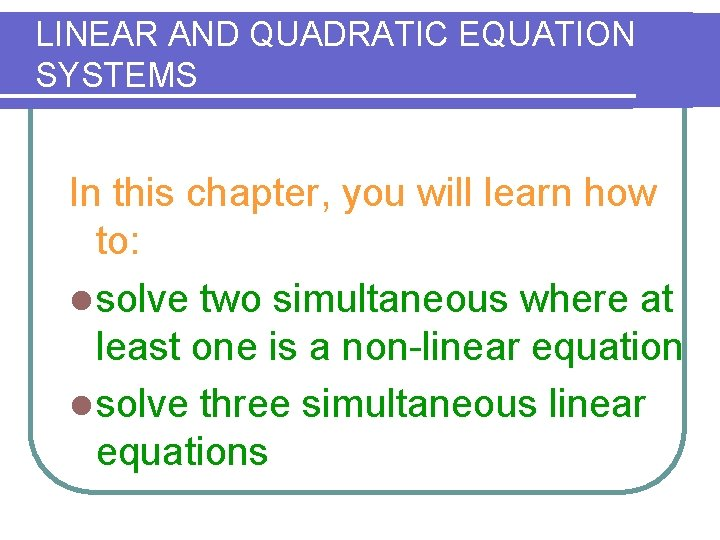LINEAR AND QUADRATIC EQUATION SYSTEMS In this chapter, you will learn how to: l solve two simultaneous where at least one is a non-linear equation l solve three simultaneous linear equationsLinear and quadratic equation systems Linear equation systems in two variable Linear equation systems in three variables Simultaneous equations: one linear and one quadratic Simultaneous Equations: Two Quadratics Applications ExercisesLinear equations system in two variables We have seen in Junior High school Mathematics that a set of simultaneous linear equations can be solved by either the method of: Elimination l Substitution l Combination both elimination and substitution lUSING ALGEBRAIC METHODS TO SOLVE SYSTEMS In this lesson you will study two algebraic methods for solving linear systems. The first method is called substitution. THE SUBSTITUTION METHOD 1 Solve one of the equations for one of its variables. 2 Substitute expression from Step 1 into other equation and solve for other variable. 3 Substitute value from Step 2 into revised equation from Step 1. Solve.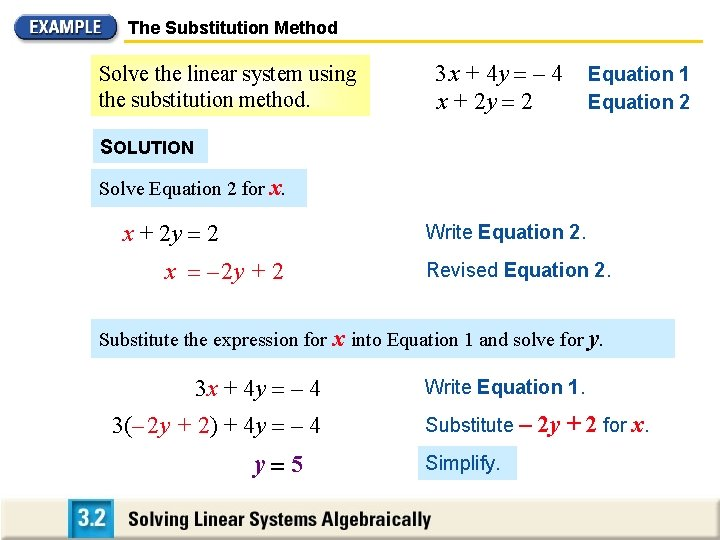The Substitution Method Solve the linear system using the substitution method. 3 x + 4 y – 4 x + 2 y 2 Equation 1 Equation 2 SOLUTION Solve Equation 2 for x. x + 2 y 2 Write Equation 2. x – 2 y + 2 Revised Equation 2. Substitute the expression for x into Equation 1 and solve for y. 3 x + 4 y – 4 3(– 2 y + 2) + 4 y – 4 y 5 Write Equation 1. Substitute – Simplify. 2 y + 2 for x.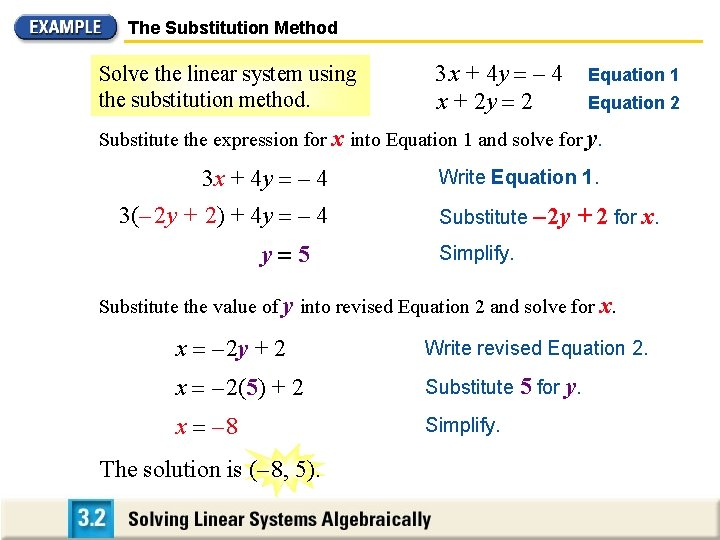The Substitution Method Solve the linear system using the substitution method. 3 x + 4 y – 4 x + 2 y 2 Equation 1 Equation 2 Substitute the expression for x into Equation 1 and solve for y. 3 x + 4 y – 4 3(– 2 y + 2) + 4 y – 4 y 5 Write Equation 1. Substitute – 2 y + 2 for x. Simplify. Substitute the value of y into revised Equation 2 and solve for x. x – 2 y + 2 Write revised Equation 2. x – 2(5) + 2 Substitute 5 for y. x – 8 Simplify. The solution is (– 8, 5).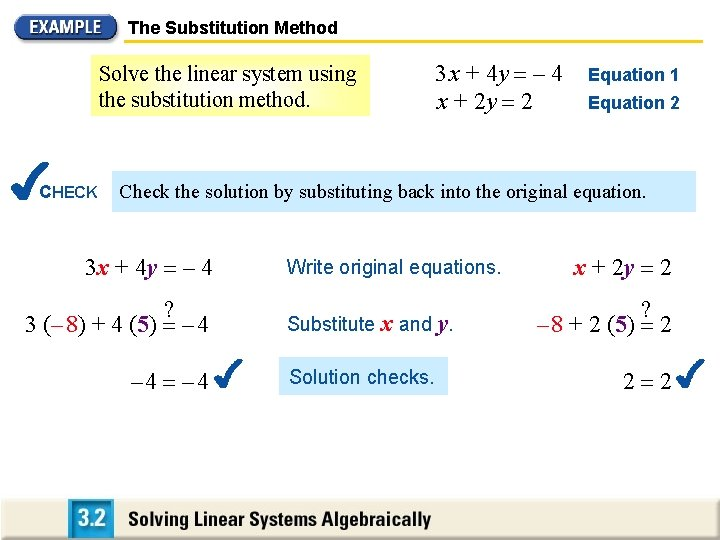The Substitution Method Solve the linear system using the substitution method. CHECK 3 x + 4 y – 4 x + 2 y 2 Equation 1 Equation 2 Check the solution by substituting back into the original equation. 3 x + 4 y – 4 ? 3 (– 8) + 4 (5) – 4 Write original equations. Substitute x and y. Solution checks. x + 2 y 2 ? – 8 + 2 (5) 2 2 2USING ALGEBRAIC METHODS TO SOLVE SYSTEMS In the first step of the previous example, you could have solved for either x or y in either Equation 1 or Equation 2. It was easiest to solve for x in Equation 2 because the x-coefficient was 1. In general you should solve for a variable whose coefficient is 1 or – 1. CHOOSING A METHOD If neither variable has a coefficient of 1 or – 1, you can still use substitution. In such cases, however, the linear combination method may be better. The goal of this method is to add the equations to obtain an equation in one variable.USING ALGEBRAIC METHODS TO SOLVE SYSTEMS THE LINEAR COMBINATION METHOD 1 Multiply one or both equations by a constant to obtain coefficients that d iffer only in sign for one of the variables. 2 Add revised eq uations from Step 1. Combine like terms to eliminate one of the variables. Solve for remaining variable. 3 Substitute value obtained in Step 2 into either original equation and solve for other variable.The Linear Combination Method: Multiplying One Equation Solve the linear system using the linear combination method. 2 x – 4 y 13 4 x – 5 y 8 Equation 1 Equation 2 SOLUTION Multiply the first equation by – 2 so that x-coefficients differ only in sign. 2 x – 4 y 13 • – 2 4 x – 5 y 8 Add the revised equations and solve for y. – 4 x + 8 y – 26 4 x – 5 y 8 3 y – 18 y – 6The Linear Combination Method: Multiplying One Equation 2 x – 4 y 13 4 x – 5 y 8 Solve the linear system using the linear combination method. Add the revised equations and solve for y. Equation 1 Equation 2 y – 6 Substitute the value of y into one of the original equations. CHECK Write Equation 1. 2 x – 4 y 13 You can check the solution algebraically using the method shown in the previous example. Substitute – 6 for y. 2 x – 4(– 6) 13 2 x + 24 13 x – The solution is ( – 11 2 Simplify. 11 2 Solve for x. ) , – 6.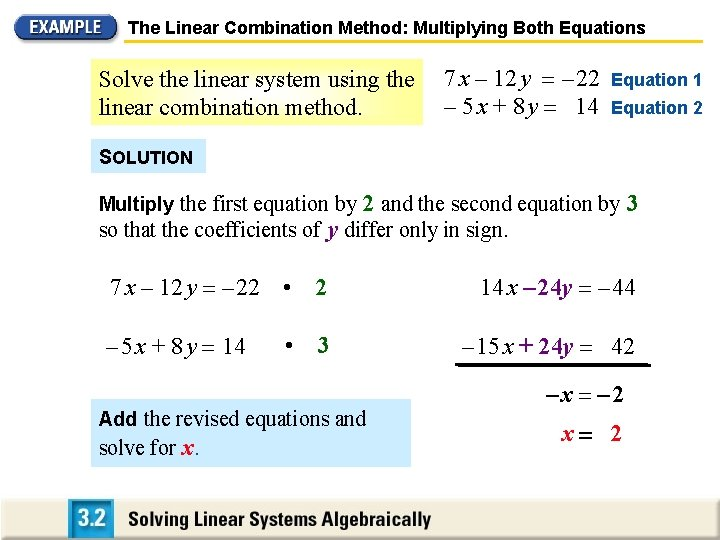The Linear Combination Method: Multiplying Both Equations Solve the linear system using the linear combination method. 7 x – 12 y – 22 – 5 x + 8 y 14 Equation 1 Equation 2 SOLUTION Multiply the first equation by 2 and the second equation by 3 so that the coefficients of y differ only in sign. 7 x – 12 y – 22 • 2 14 x – 24 y – 44 – 5 x + 8 y 14 3 – 15 x + 24 y 42 • Add the revised equations and solve for x. –x – 2 x 2The Linear Combination Method: Multiplying Both Equations Solve the linear system using the linear combination method. 7 x – 12 y – 22 – 5 x + 8 y 14 Add the revised equations and solve for x. Equation 1 Equation 2 x 2 Substitute the value of x into one of the original equations. Solve for y. – 5 x + 8 y 14 – 5 (2) + 8 y 14 y=3 Write Equation 2. Substitute 2 for x. Solve for y. The solution is (2, 3). Check the solution algebraically or graphically.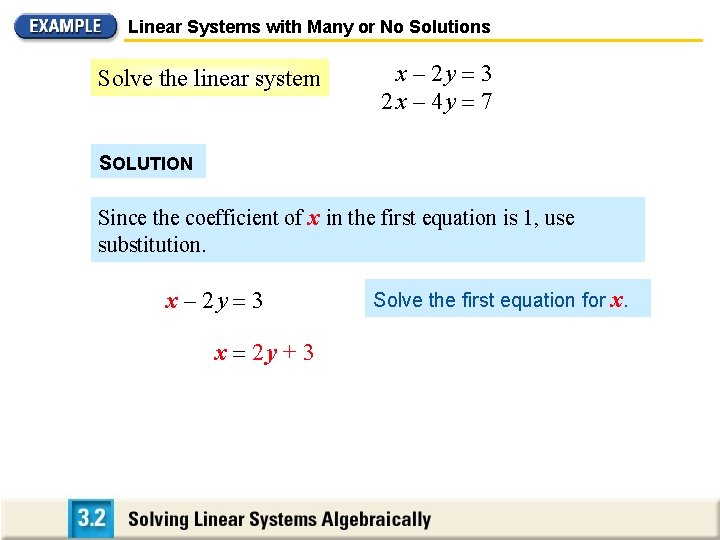Linear Systems with Many or No Solutions Solve the linear system x – 2 y 3 2 x – 4 y 7 SOLUTION Since the coefficient of x in the first equation is 1, use substitution. x – 2 y 3 x 2 y + 3 Solve the first equation for x.Linear Systems with Many or No Solutions Solve the linear system x 2 y + 3 x – 2 y 3 2 x – 4 y 7 Solve the first equation for x. Substitute the expression for x into the second equation. 2 x – 4 y 7 2(2 y + 3) – 4 y 7 6 7 Write second equation. Substitute 2 y + 3 for x. Simplify. Because the statement 6 = 7 is never true, there is no solution.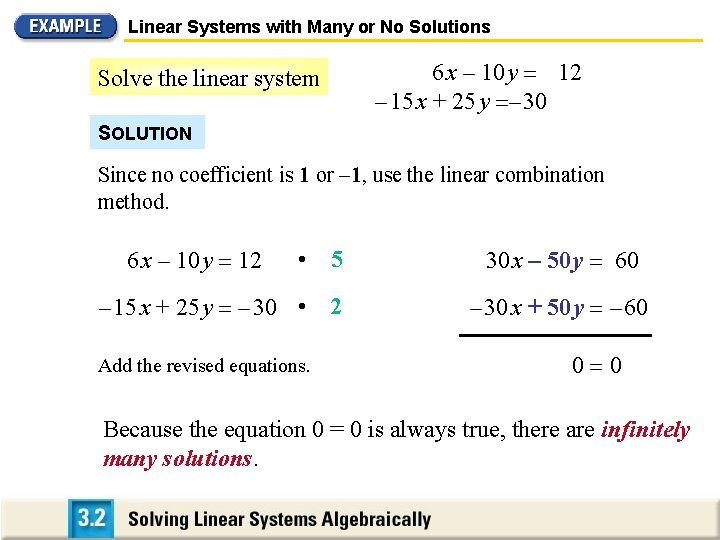Linear Systems with Many or No Solutions 6 x – 10 y 12 – 15 x + 25 y – 30 Solve the linear system SOLUTION Since no coefficient is 1 or – 1, use the linear combination method. 6 x – 10 y 12 • 5 30 x – 50 y 60 – 15 x + 25 y – 30 • 2 – 30 x + 50 y – 60 Add the revised equations. 0 0 Because the equation 0 = 0 is always true, there are infinitely many solutions.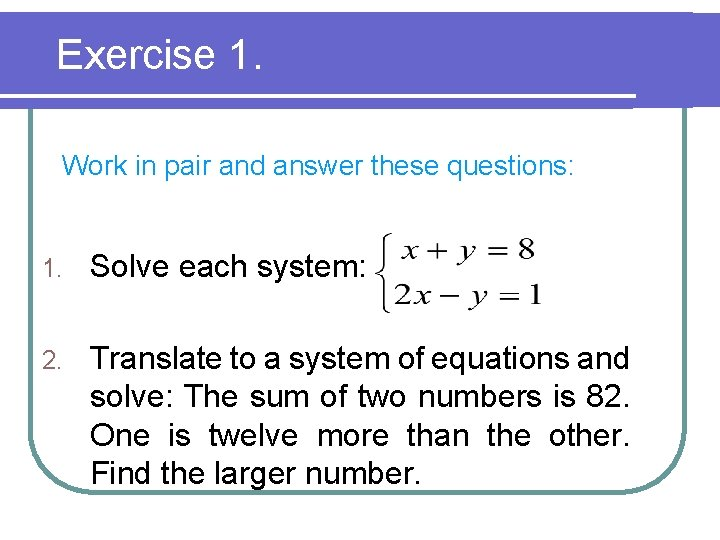Exercise 1. Work in pair and answer these questions: 1. Solve each system: 2. Translate to a system of equations and solve: The sum of two numbers is 82. One is twelve more than the other. Find the larger number.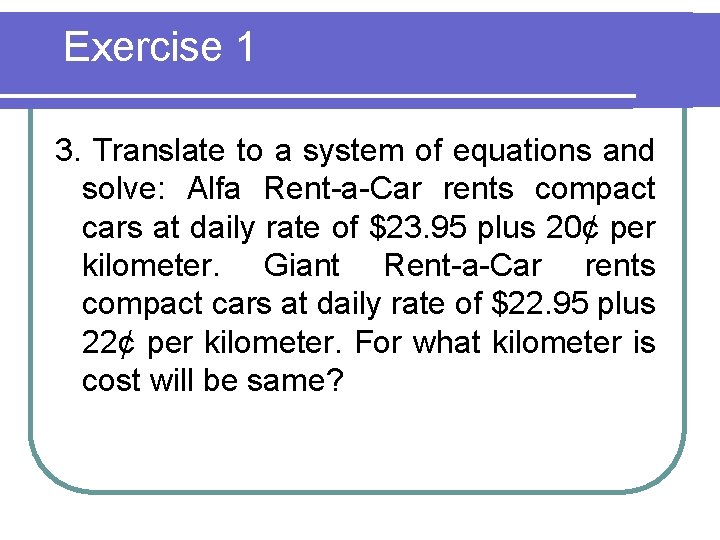Exercise 1 3. Translate to a system of equations and solve: Alfa Rent-a-Car rents compact cars at daily rate of \$23. 95 plus 20¢ per kilometer. Giant Rent-a-Car rents compact cars at daily rate of \$22. 95 plus 22¢ per kilometer. For what kilometer is cost will be same?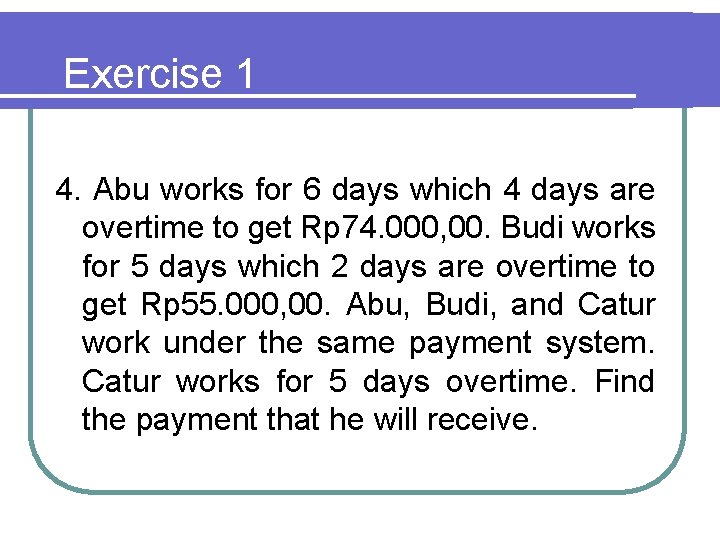Exercise 1 4. Abu works for 6 days which 4 days are overtime to get Rp 74. 000, 00. Budi works for 5 days which 2 days are overtime to get Rp 55. 000, 00. Abu, Budi, and Catur work under the same payment system. Catur works for 5 days overtime. Find the payment that he will receive.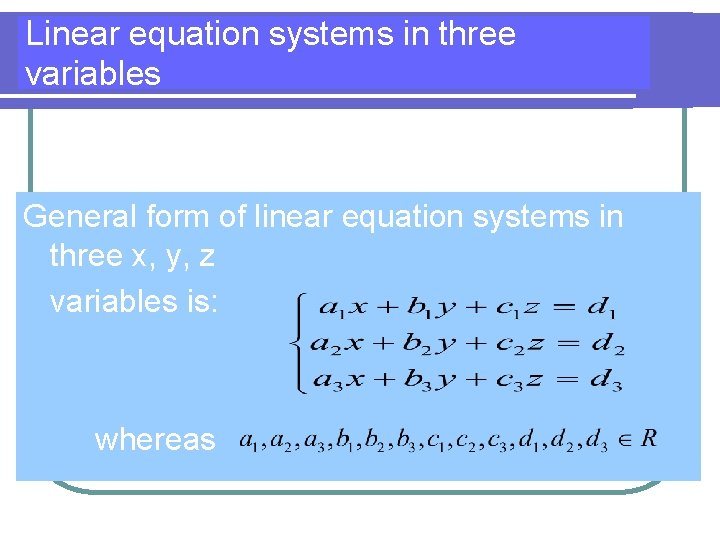Linear equation systems in three variables General form of linear equation systems in three x, y, z variables is: whereasAnswering the linear equation system with three variables is determining that is a simultaneous solution from the equation system, so the solution set is: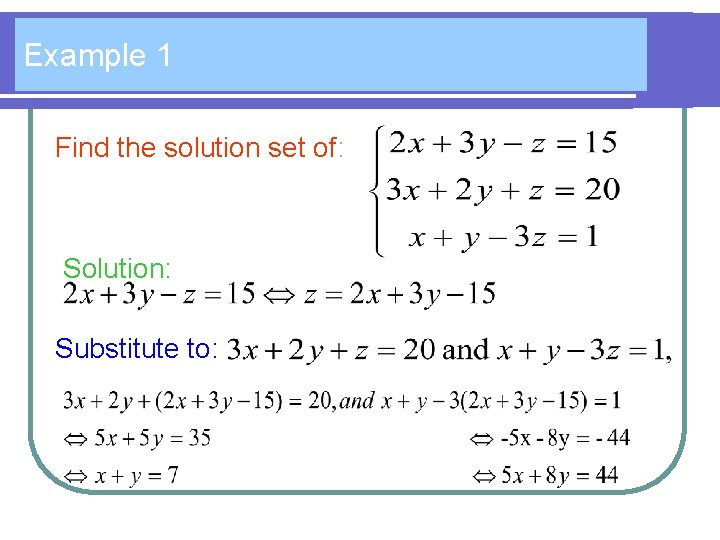Example 1 Find the solution set of: Solution: Substitute to:Then we will find the linear equation system: And it is substituted into: 5 x + 8 y = 4 4 we will find: And put the result into: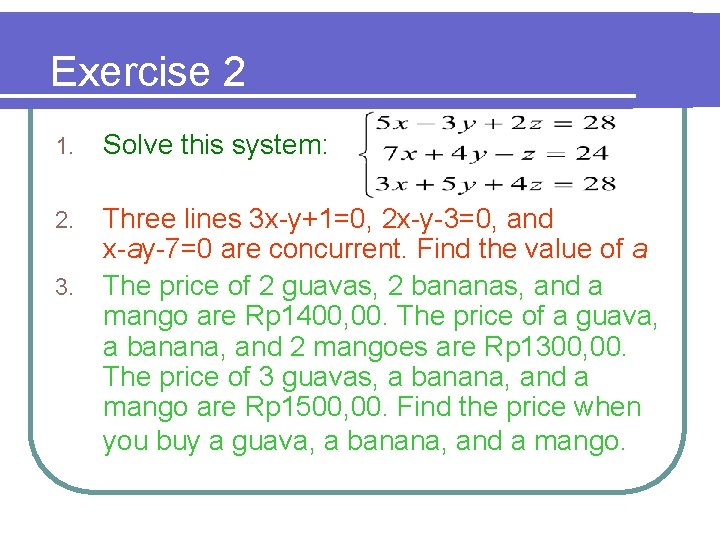Exercise 2 1. Solve this system: 2. Three lines 3 x-y+1=0, 2 x-y-3=0, and x-ay-7=0 are concurrent. Find the value of a The price of 2 guavas, 2 bananas, and a mango are Rp 1400, 00. The price of a guava, a banana, and 2 mangoes are Rp 1300, 00. The price of 3 guavas, a banana, and a mango are Rp 1500, 00. Find the price when you buy a guava, a banana, and a mango. 3.Exercise 2 4. Do the exercises in: … p. …. no…. , p. …. number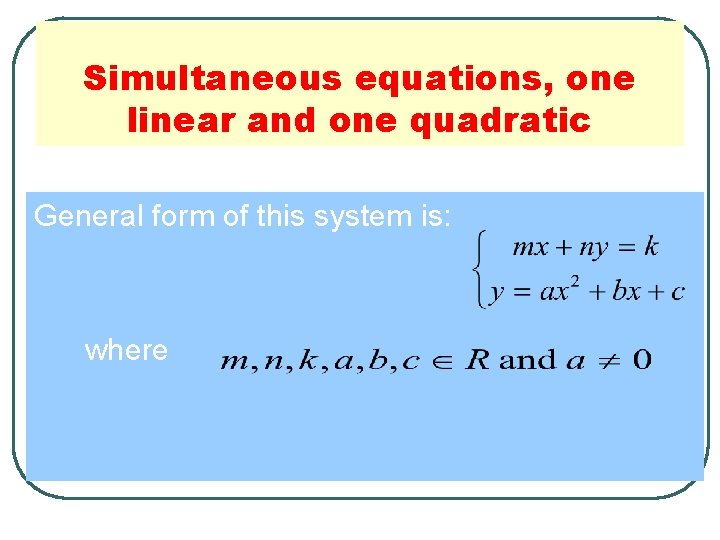Simultaneous equations, one linear and one quadratic General form of this system is: whereSolve the following system: (1) (2) Solution: From the linear equation : y = 3 x-1 substitute to (2) (4 x-1)(x-2)=0 x = ¼ or x =2 The solution is either x = ¼ => y = - ¼ or x = 2=> y = 5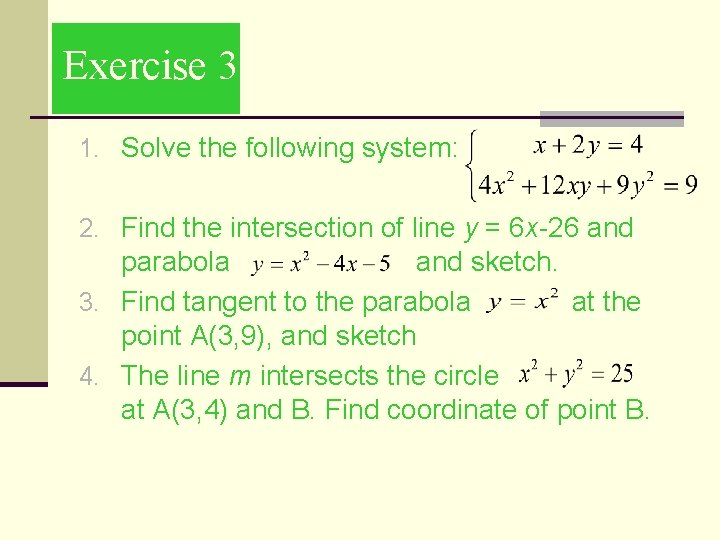Exercise 3 1. Solve the following system: 2. Find the intersection of line y = 6 x-26 and parabola and sketch. 3. Find tangent to the parabola at the point A(3, 9), and sketch 4. The line m intersects the circle at A(3, 4) and B. Find coordinate of point B.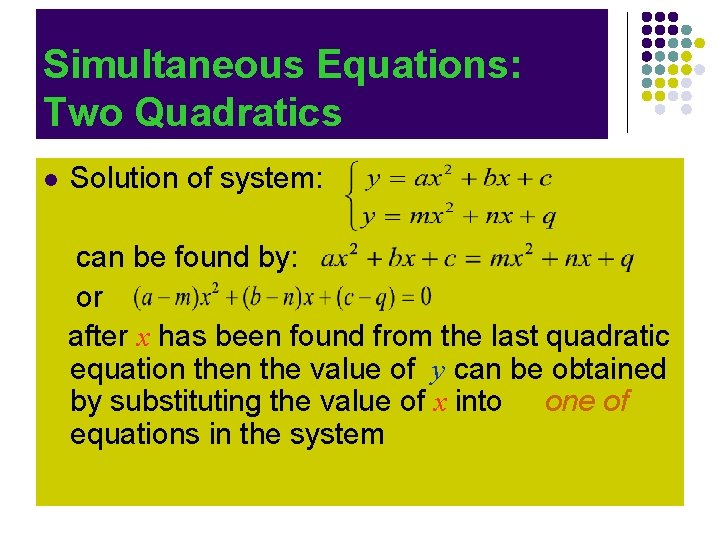Simultaneous Equations: Two Quadratics l Solution of system: can be found by: or after x has been found from the last quadratic equation the value of y can be obtained by substituting the value of x into one of equations in the system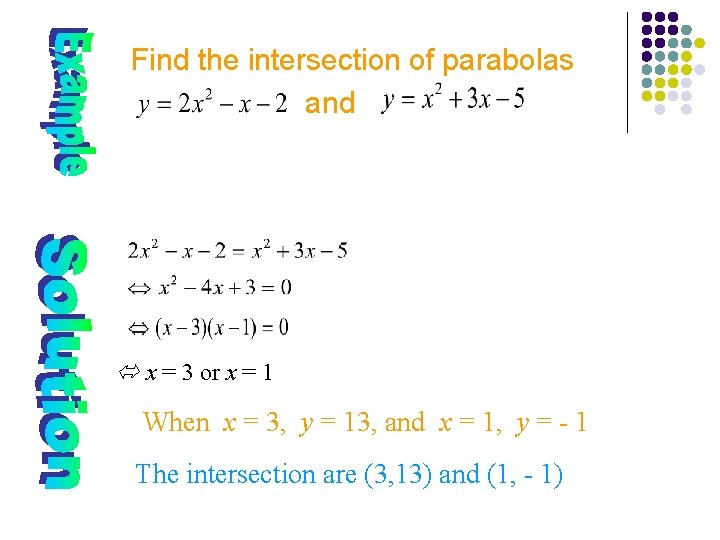Find the intersection of parabolas and x = 3 or x = 1 When x = 3, y = 13, and x = 1, y = - 1 The intersection are (3, 13) and (1, - 1)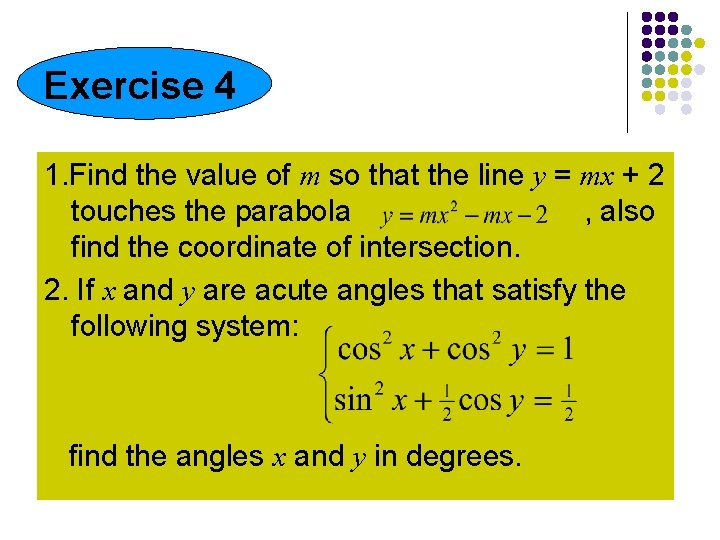Exercise 4 1. Find the value of m so that the line y = mx + 2 touches the parabola , also find the coordinate of intersection. 2. If x and y are acute angles that satisfy the following system: find the angles x and y in degrees.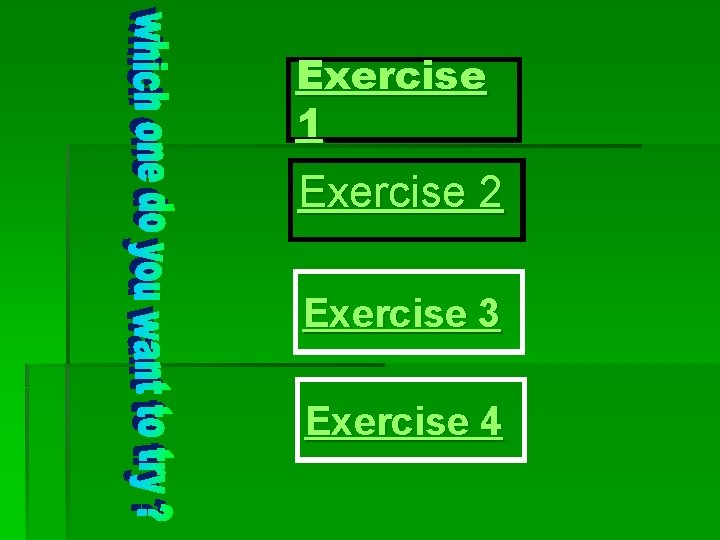Exercise 1 Exercise 2 Exercise 3 Exercise 4Simultaneous equations: one is linear and the other one is quadratic General form of this system is: whereas